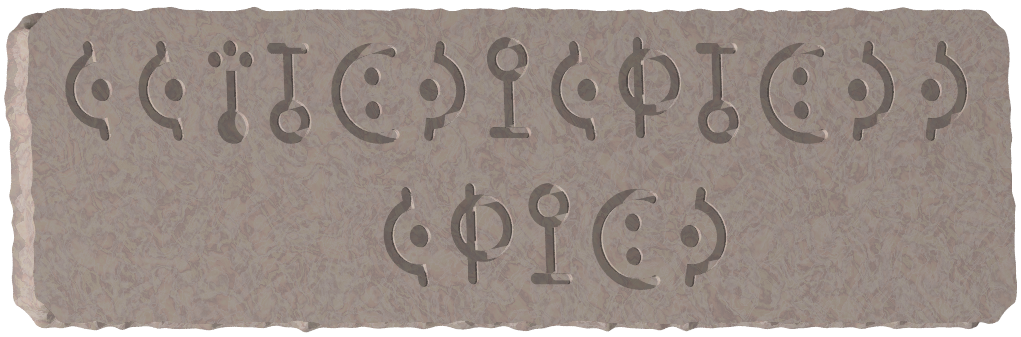Time Limit : sec, Memory Limit : KB

### Icpcan AlphabetResearch on the civilization of old kingdom of Icpca has been carried out at the Investigation Center for Prehistoric Civilization (ICPC). Their recent excavation found two expressions engraved on a stone wall at the ruins of Icpca. The expressions are composed of n distinct Icpca letters a1, ..., an, symbols corresponding to inequality signs ('<' and '>') and parentheses ('(' and ')'). In what follows, they are represented by capital Roman letters and ordinary symbols for description ease. Expressions conform to the following grammar rules with the start symbol E.

  E ::= F | '(' E '<' E ')' | '(' E '>' E ')'
F ::= a1 | a2 | ... | an


Analyses unifying multifarious knowledge on the old kingdom and its civilization revealed the following evaluation rules.

• A total order relation is defined on the set of letters a1, ..., an.
• When an expression consists only of a single letter, the value of the expression is that very letter.
• For two expressions P and Q with values ai and aj, respectively, the value of the expression (P<Q) is one of the letters ai or aj that precedes in the total order. If the two letters are the same, the value is that letter.
• Similarly, for two expressions P and Q with values ai and aj, respectively, the value of the expression (P>Q) is one of the letters ai or aj that comes later in the total order. If the two letters are the same, the value is that letter.

It was also found that the values of the two expressions discovered must be equal. How many different total orders, among n! possible total orders, make the values of the two expressions equal?

### Input

The input consists of multiple datasets, each in the following format.

n
a1a2...an
S
T

Each dataset consists of four lines. The first line contains n (1 ≤ n ≤ 16), the number of different capital Roman letters corresponding to Icpcan characters. The second line contains n distinct capital Roman letters without any spaces. The third and the fourth lines contain two discovered expressions S and T, respectively. Both S and T conform to the grammar given above and neither of them has more than 100 characters.

The end of the input is indicated by a line containing a zero. The number of datasets does not exceed 50.

### Output

For each dataset, output a single line containing the number of different total orders making the values of the two expressions equal.

### Sample Input

3
CIP
((I<C)>(P<C))
(P>C)
2
AB
(A<B)
(A>B)
3
ANY
((A<N)<Y)
((N<Y)<((A<A)<N))
6
A
((E>(A>((E<W)>(N<S))))>(A<W))
0


### Output for the Sample Input

1
0
6
300